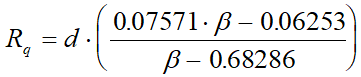Calculation of Flow rate - Intergraph Smart Instrumentation - 13.1 - Reference - Hexagon PPM

Integraph Smart Instrumentation Sizing Equations

Language
English (United States)
Product
Intergraph Smart Instrumentation
Search by Category
Reference
Smart Instrumentation Version
13.1
1. Calculate internal pipe diameter at flow temperature: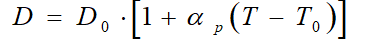2. Calculate correction factor for steam quality (for liquid, water, and gas FS=1);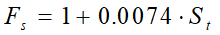3. Calculate orifice diameter at flow temperature.

1. For Lo-Loss tube, nozzles,and Venturi tubes: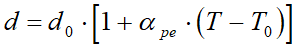2. For other orifices: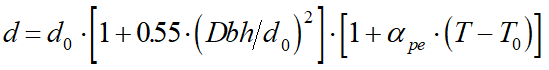4. Calculate b ratio.

1. For all Flow meters except Segmental orifices: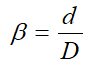2. Segmental orifices.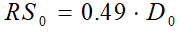2. Calculate b ratio.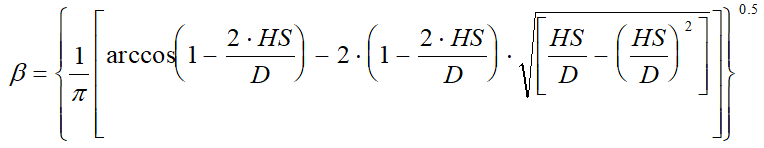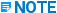HS is calculated with the help of HS0 in accordance with step 3b.

5. Calculate gas expansion factor (for liquid and water, EPS=1).

1. For square edge orifice with 2½D and 8D pipe taps: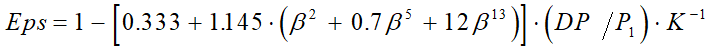2. For Lo-Loss tube, nozzles,and Venturi tubes: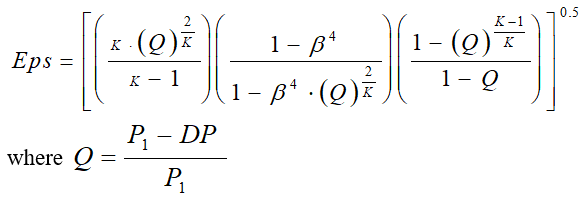3. For eccentric orifices: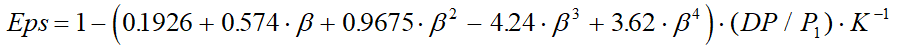4. For square-edge orifices specified in ISO 5167-2003 (flange tappings, corner tappings, and radius tappings):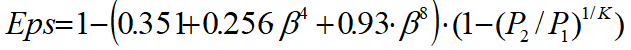5. For other Flow meters: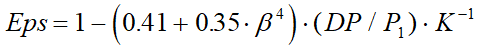6. Calculate first estimate for flow rate:

*W0 [kg/s]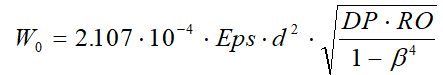7. Calculate Pipe Reynolds number:

*W0 [kg/s]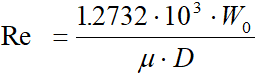9. Calculate new flow rate:

*W[kg/s]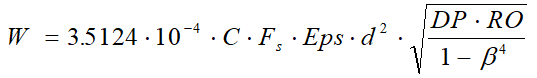10. For quarter of circle (Quadrant Edge) orifice, Lo-loss tube, Venturi tubes, and Segmental orifices go to step 13.

11. Calculate the relative change in Flow rate: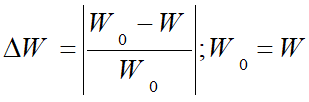12. Repeat steps 7 to 11 until the relative change in the flow rate is less than 0.0001.

13. For quarter of circle (Quadrant Edge) orifice, calculate radius of upstream profile: